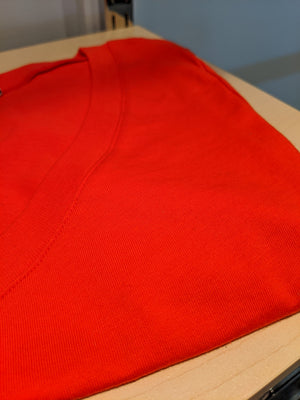oui

# V Neck Tee

Regular price \$85 now \$59.50 Unit price  per

Shipping calculated at checkout.
• 100% cotton
• Simple v nck tee
• Short sleeve
• Loose fit
• Can be worn all year round

SIZE GUIDE

• 34 = 4 = Small
• 36 = 6 = Small
• 38 = 8 = Medium
• 40 = 10 = Medium
• 42 = 12 = Large
• 44 = 14 = Large
• 46 = 16 = X-Large